Courses

# Examples with Solutions - Human Eye and Colourful World Class 10 Notes | EduRev

## Class 10 : Examples with Solutions - Human Eye and Colourful World Class 10 Notes | EduRev

The document Examples with Solutions - Human Eye and Colourful World Class 10 Notes | EduRev is a part of the Class 10 Course Science Class 10.
All you need of Class 10 at this link: Class 10

Q1. A lens has power of - 2.5 D. What is the focal length and nature of the lens?
Sol. P = - 2.5D, f = ?

From relation, P = 1/f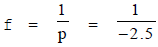= - 0.4 m = - 40 cm

Negative sign indicates that it is a concave lens.

Q2. A doctor has prescribed a corrective lens of power + 1.5 D. Find the focal length of the lens. Is the prescribed lens diverging or converiging?
Sol. p = + 1.5 D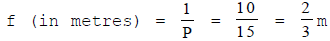= 66.6 cm

As the focal length and power of the lens is positive therefore, lens is a convex (converging lens).

Q3. A person with a myopic eye cannot see beyond 1.2 m distinctly. What should be the nature of the corrective lens used to restore proper vision?
Sol. Corrective lens required is 'concave lens' of suitable power to restore proper vision. In this cases,

u = -∞, v = -1.2m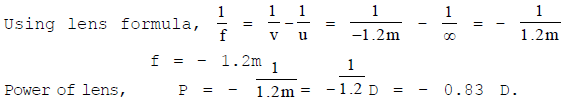Q4. An object 5 cm in length is held 25 cm away from a converging lens of focal length 10 cm. Draw the ray diagram and find the position, size and the nature of the image formed.
Sol.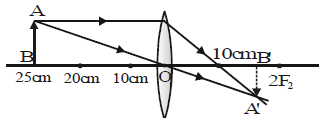f = + 10 cm

u = -25 cm

Using lens formula,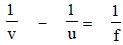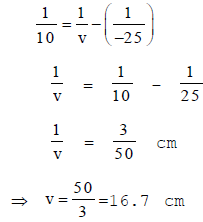The image is real at a distance of 16.7 cm behind the lens.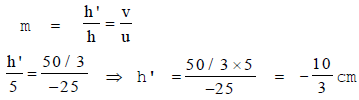Height of the image is 3.3 cm in height.
Q5. The refractive index of diamond is 2.47 and that of glass is 1.51. How much faster does light travel in glass than in diamond?
Sol. Let n1 and n2 be the refractive indices and v1 and v2 be the velocity of light in diamond and glass respectively, then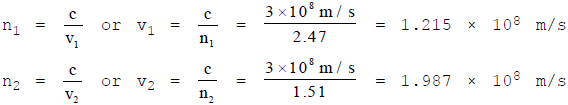v2 - v1 = (1.987 - 1.215) x 108 = 0.772 x 108 m/s

= 7.72 x 107 m/s

Thus light travels 7.72 x 107 m/s faster in glass than diamond.

Q6. A magnifying lens has a focal length of 10 cm.
(a) Where should the object be placed if the image is to be 30 cm from the lens ?
(b) What will be the magnification ?
Sol. (a) In case of magnifying lens, the lens is convergent and the image is erect, enlarged, virtual, between infinity and object and on the same side of lens.

f = 10 cm and v = - 30 cm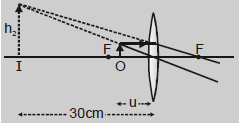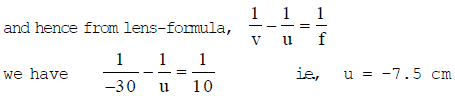So the object must be placed in front of lens at a distance of 7.5 cm (which is < f) from it.

(b)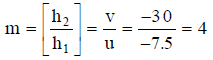i.e., image is erect, virtual and four times the size of object.

Q7. An object 25 cm high is placed in front of a convex lens of focal length 30 cm. If the height of image formed is 50 cm, find the distance between the object and the image ?
Sol. As object is in front of the lens, it is real and as

h1 = 25 cm, f = 30 cm, h2 = - 50 cm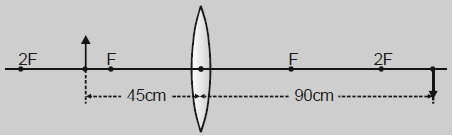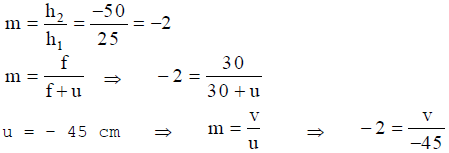⇒ v = 90 cm

As in this situation, object and image are on opposite sides of lens, the distance between object and image is given by-

d1 = u + v = 45 + 90 = 135 cm

If the image is erect (i.e., virtual)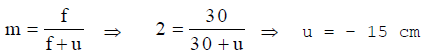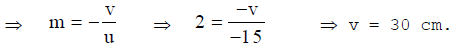As in this situation, both image and object are in front of the lens, the distance between object and image is given by-

d2 = v - u = 30 - 15 = 15 cm.

Q8. A needle placed 45 cm from a lens forms an image on a screen placed 90 cm on other side of the lens. Identify the type of lens and determine its focal length, What is the size of the image, if the size of the needle is 5 cm?
Sol. Here, u = -45 cm, v = 90 cm, f = ?, h2 = ?, h1 = 5 cm,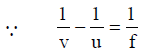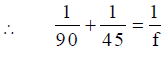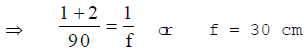As f is positive, the lens is coverging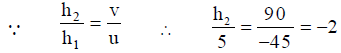⇒ h2 = -10 cm.

Minus sign indicates that image is real and inverted

Q9. A beam of light converges to a point P. A lens is placed in the path of the convergent beam 12 cm from P. At what point does the beam converge if the lens is
(a) a convex lens of focal length 20 cm.
(b) a concave lens of focal length 16 cm.
Sol. Here, the point P on the right of the lens acts as a virtual object,

u = 12 cm, v = ?

(a) f = 20 cm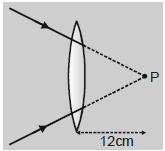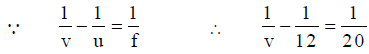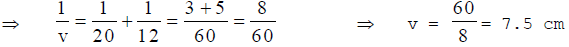(b) f = -16 cm, u = 12 cm,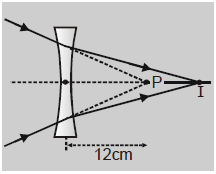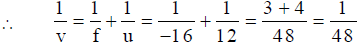⇒ v = 48 cm

Hence image is at 48 cm to the right of the lens, where the beam would converge.

Q10. An object is placed at a distant of 1.50 m from a screen and a convex lens placed in between produces an image magnified 4 times on the screen. What is the focal length and the position of the lens.
Sol.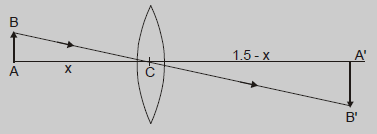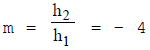Let the lens be placed at a distance of x from the object.

Then u = – x, and v = (1.5 – x)

using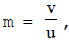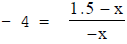⇒ x = 0.3 metre

The lens is placed at a distance of 0.3 m from the object (or 1.20 m from the screen)

For focal length, we may use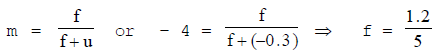= 0.24 cm

Q11. One-half of a convex lens is covered with a black paper. Will this lens produce a complete image of the object? Verify your answer experimentally. Explain your observations.
Sol. Yes, it wil produce a complete image of the object, as shown in fig. This can be verified experimentally by observing the image of a distance object like tree on a screen, when lower half of the lens is covered with a black paper. However, the intensity of brightness of image will reduce.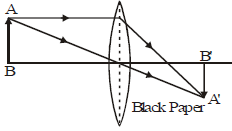Q12. An object is placed at a distance of 10 cm from a convex mirror of focal length 15 cm. Find the position and nature of the image.
Sol. Here, object distance, u = - 10 cm focal length, f = 15 cm, Image distance, v = ?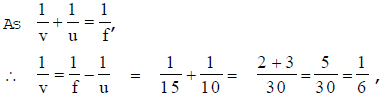v = 6 cm

Here, + sign of v indicates that image is at the back of the mirror. It must be virtual, erect and smaller in size than the object.
Q13. The magnification produced by a plane mirror is +1. What does this mean?  [NCERT]
Sol. As m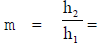+ 1, h2 = h1

i.e., size of image is equal to size of the object. Further, + sign of m indicates that the image is erect and hence virtual.

Q14. Find the focal length of a lens of power - 2.0 D. What type of lens is this? [NCERT]
Sol. Here, focal length f = ?, power P = - 2.0 D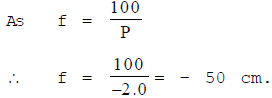Q15. The far point of a myopic person is 80 cm in front of the eye. What is the nature and power of the lens required to correct the problem?
Sol. Distance of far point, x = 80 cm, P = ?

For viewing distant objects, focal length of corrective lens,

f = - x = 80 cm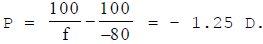The lens is concave.

Q16. Why is a normal eye not able to see clearly the objects placed closer than 25 cm?
Sol. This is because the focal length of eye lens cannot be decreased below a certain minimum limit.

Offer running on EduRev: Apply code STAYHOME200 to get INR 200 off on our premium plan EduRev Infinity!

## Science Class 10

85 videos|255 docs|129 tests

,

,

,

,

,

,

,

,

,

,

,

,

,

,

,

,

,

,

,

,

,

;